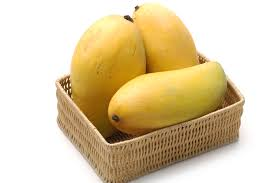# I am a mango -_-

Probability Level 3If you are given $\color{#20A900}{\textbf{18 raw mangoes}}$, $\color{#D61F06}{\textbf{14 ripe mangoes}}$ and $\color{#624F41}{\textbf{10 rotten mangoes}}$, how many different groups of $20$ mangoes can you form such that there are at least $5$ mangoes of each type ?

$\bullet$ This problem is a part of the set Vegetable combinatorics

×